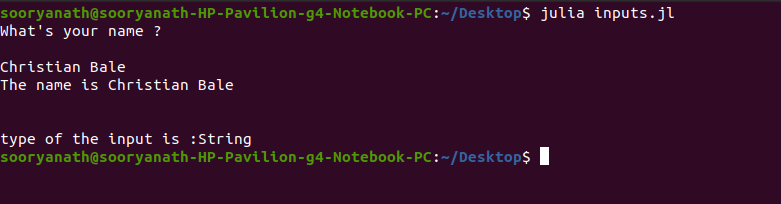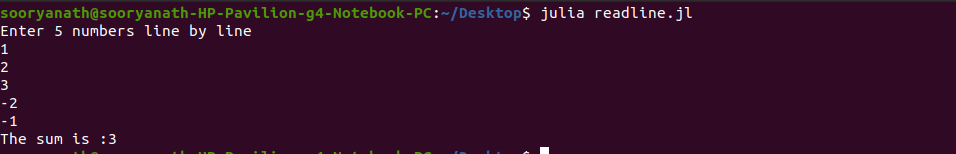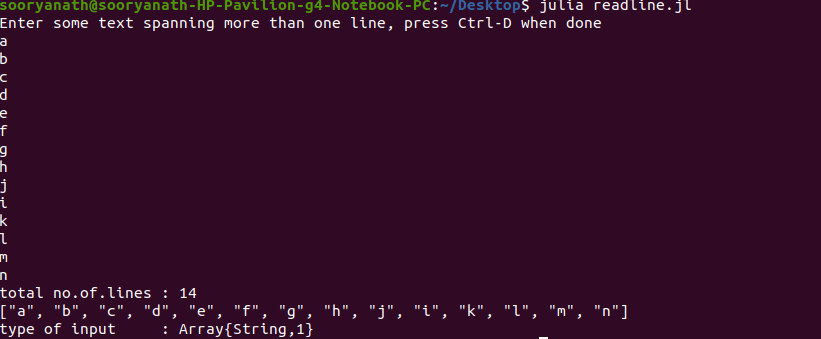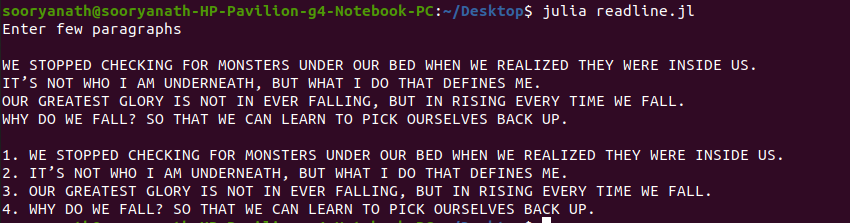# Taking Input from Users in Julia

Reading user input is a way of interaction between the program and the user. User inputs are necessary in case of testing a piece of code with varying and legitimate inputs.

Reading user inputs from console in Julia can be done through inbuilt I/O methods like :

`readline()` method reads a line of text from the input stream (STDIN) until a ‘\n’ (newline) character is encountered. The input is read as String data.

Syntax:

```s = readline()
```

Example 1:
In the below example, user input is obtained using the `readline()` method. We can prompt the user with the help of a print statement prior. It is to be noted that readline method reads the input into a string data type.

 `# Julia program to take input from user ` ` `  `# prompt to input ` `print``(``"What's your name ? \n\n"``)  ` ` `  `# Calling rdeadline() function ` `name ``=` `readline() ` ` `  `println(``"The name is "``, name) ` `print``(``"\n\n"``) ` ` `  `# typeof() determines the datatype. ` `println(``"Type of the input is: "``, typeof(name))  `

Output :Example 2: Reading numerical data types from console

The below example demonstrates how to read inputs as numbers and make use of them in further computations. This is done using the `parse()` method, using which we can convert a numeric string(Float or Int) into a numerical value.

 `# Julia program to calculate sum of  ` `# 5 integers obtained from console/user input ` ` `  `result ``=` `0` ` `  `# Prompt to enter ` `println(``"Enter 5 numbers line by line"``) ` ` `  `# Taking Input from user ` `for` `number ``in` `1``:``5`  ` `  `   ``num ``=` `readline() ` `   ``num ``=` `parse(Int64, num)  ` `   ``global` `result``+``=` `num   ` ` `  `end ` ` `  `println(``"The sum is :"``, result) `

Output:`readlines()` method is used to read N lines of text input from the console. N lines are stored as the entries of a one-dimensional String array. Here lines must be delimited with newline character or by pressing the “ENTER” key. To stop taking Input press Ctrl-D.

Syntax:

```lines = readlines()
```

Example 1: Reading N lines of input from STDIN
In the below example N-lines of input are obtained and stored within a 1-D String Array. We can access the desired line using it’s index.

 `# Julia program to take  ` `# multi-lined input from user ` ` `  `line_count ``=` `0` ` `  `println(``"Enter multi-lined text, press Ctrl-D when done"``) ` ` `  `# Calling readlines() function ` `lines ``=` `readlines() ` ` `  `# Loop to count lines ` `for` `line ``in` `lines ` `   `  `   ``global` `line_count ``+``=` `1` `  `  `end ` ` `  `println(``"total no.of.lines : "``, line_count) ` ` `  `println(lines) ` ` `  `# Getting type of Input values ` `println(``"type of input: "``, typeof(lines)) `

Output:### Example 2

In the below example we input N lines and display the entries by prefixing with their entry numbers.

 `line_number ``=` `1` ` `  `println(``"Enter few paragraphs"``) ` ` `  `print``(``"\n"``) ` ` `  `lines ``=` `readlines() ` ` `  `println() ` ` `  `for` `line ``in` `lines ` `   `  `   ``println(line_number, ``". "``, line) ` `   ``global` `line_number ``+``=` `1` `  `  `end `

Output:Whether you're preparing for your first job interview or aiming to upskill in this ever-evolving tech landscape, GeeksforGeeks Courses are your key to success. We provide top-quality content at affordable prices, all geared towards accelerating your growth in a time-bound manner. Join the millions we've already empowered, and we're here to do the same for you. Don't miss out - check it out now!